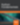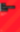# jQuery Matrix Effects

JavaScript

Share:

#### Free JavaScript Book!Write powerful, clean and maintainable JavaScript.

RRP \$11.95

## Introduction

This is a cool jQuery Plugin that is based on the famous film “Matrix”. Well this is pretty straightforward. This plugin imitates the greenie with number thing effects from the film itself, choose an image that will turn into it and start doing modifications. You should try this, its fun!## How does it work?

When you load image into canvas, it’s possible to read the pixels and then colors:

``data =  ctx.getImageData(x, y, settings.grainSize, settings.grainSize).data``

The exciting part is that it’s possible to read rectangles of the image, jQuery uses this to calculate average color of every portion of the image. Then, that color will be used by the character that will replace the pixels of an image portion.

``````//get a portion of image
data = ctx.getImageData(x, y, settings.grainSize, settings.grainSize).data;
var r_avg = 0, g_avg = 0, b_avg = 0;

//sum all channels values
for (var i = 0; i &lt; data.length; i += 4) {
r_avg += data[i];
g_avg += data[i + 1];
b_avg += data[i + 2];
}

//calculate average color for each channel
r_avg = Math.round(r_avg / (data.length / 4));
g_avg = Math.round(g_avg / (data.length / 4));
b_avg = Math.round(b_avg / (data.length / 4));``````

It’ll generate lots of B tags, each have character inside:

``````var block = document.createElement("b");
jQuery(matrix_cont).append(block);
var r = image_colors[i].r;
var g = image_colors[i].g;
var b = image_colors[i].b;
block.innerHTML = getChar(r, g, b);
switch (settings.colors) {
case "bn":
var gray = Math.round((r + g + b) / 3);
jQuery(block).css("color", "rgb(" + gray + "," + gray + "," + gray + ")");
break;
case "green":
jQuery(block).css("color", "rgb(0," + g + ",0)");
break;
case "all":
default:
jQuery(block).css("color", "rgb(" + r + "," + g + "," + b + ")");
break;
}``````

Knowing the characters
First characters are for the dark colors, while last are for the light.

``````chars: ['.', '¸', '¹', '`', '*', '_', '°', 'ª', '^', '+', '±', '¢', '®', '"', 'υ',
'»', '½', '¾', 'h', 'e', '8', 's', 'p', '=', '/', '\$', '§', 'ξ', 'u', '6', '9',
'5', 'y', 'j', 'd', 'q', 'H', 'ç', 'B', 'V', '8', 'Z', 'W', 'S',
'%', 'e', 'n', 'm', '&amp;', 'à', 'ω', 'Ψ', 'o', '#', 'k', '●', '♦', '♥']``````

## How to use

``````<!--mce:0-->
<!--mce:1-->``````

And use this one to activate it.

``\$(function () { \$("img").matrix(); });``### Sam Deering

Sam Deering has 15+ years of programming and website development experience. He was a website consultant at Console, ABC News, Flight Centre, Sapient Nitro, and the QLD Government and runs a tech blog with over 1 million views per month. Currently, Sam is the Founder of Crypto News, Australia.

#### New books out now!Learn valuable skills with a practical introduction to Python programming!Give yourself more options and write higher quality CSS with CSS Optimization Basics.